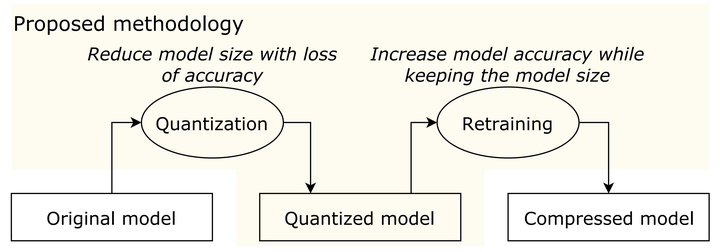# Retraining Quantized Neural Networks without Labeled DataRunning neural network models on edge devices is attracting much attention by neural network researchers since edge computing technology is becoming more powerful than ever. However, deploying large neural network models on edge devices is challenging due to the limitation in available computing resources and storage space. Therefore, model compression techniques have been recently studied to reduce the model size and fit models on resource-limited edge devices. Compressing neural network models reduces the size of a model, but also degrades the accuracy of the model since it reduces the precision of weights in the model. Consequently, a retraining method is required to recover the accuracy of compressed models. Most existing retraining methods require the original labeled training datasets to retrain the models, but labeling is a timeconsuming process. In particular, we cannot always access the original labeled datasets because of privacy policies and license limitations. In this work, we propose a method to retrain a compressed neural network model with an unlabeled dataset that is different from the original labeled dataset. We compress the model using quantization to decrease the size of the model. Subsequently, the compressed model is retrained by our proposed retraining method without using a labeled dataset to recover the accuracy of the model.

## Methodology

The proposed retraining method to recover the accuracy of quantized neural network models. The above figure shows the overview of the proposed method. We first apply quantization to the model. Subsequently, the proposed retraining method is applied to the compressed model.

Quantization is a lossy data compression technique where a range of data is grouped or binned into a single value or centroid. It is a nonlinear map that partitions the whole space and represents the values in each subspace by their centroid. Each layer in a neural network model has a different number and distribution of parameters. Thus, different number of centroids must be chosen for each individual layer. To find the best number of centroids for each layer, we quantized each layer separately and balance between the reduced model size and the accuracy loss.

After the quantization and compression processes, the compressed model is retrained to recover the accuracy without using the original labeled dataset. The below figure shows the overview of our proposed retraining method. We split the layers of the model into trainable layers and non-trainable layers. We keep the quantized layers as non-trainable layers because the unnecessary parameter values have been already eliminated in the quantized layers, and also the retraining method might be able to produce unnecessary parameter values that will just increase the model size without improving the model accuracy. We therefore choose only non-quantized layers as trainable layers for the retraining method. This approach is also helpful to shorten the retraining time since it takes longer if we handle more layers as trainable.Proposed retraining method

In our method, we leverage the outputs from the original model to retrain the quantized model instead of using the original labeled dataset. We supply the same unlabeled dataset to both the original model and the quantized model and acquire the output vectors from the two models.

## Evaluation

We evaluated the proposed method by VGG-16 and ResNet-50. These two models achieve outstanding performance in image classification tasks. The experiments were conducted using the computational resource of the AI Bridging Cloud Infrastructure (ABCI) provided by the National Institute of Advanced Industrial Science and Technology (AIST).Experimental result for VGG-16

The above figure shows the experimental result for VGG-16 Quantizing the 14th and 15th layers using 32–256 centroids achieved nearly the accuracy of the original model (75.12%). Therefore, the best configuration for quantizing the VGG-16 model is to quantize the biases in all layer using one centroid and the weights in 14th and 15th layer using 32 centroids because it compressed to possible smallest model size without significant accuracy loss.Experimental result for ResNet-50

We found that using 128 or less centroids clearly degrades the model accuracy as shown in the above figure. In addition, quantizing the 13rd–49th layers using 256 centroids, achieves the model accuracy close to the original model. Therefore, the best configuration to quantize ResNet-50 is to quantize the biases in all layer using one centroid and the weights in the 13rd–49th layers using 256 centroids.

In this work, we used VGG-16 and ResNet-50 to evaluate our proposed methodology. The model size of VGG-16 was reduced by 81.10% with only 0.34% loss of accuracy. The difference of accuracy after applying the conventional and proposed retraining was only 0.11%. ResNet-50 was reduced by 52.54% with only 0.71% loss of accuracy. The difference of accuracy after applying the conventional and proposed retraining method with only 0.03%.

## Publication

• K. Thonglek, K. Takahashi, K. Ichikawa, C. Nakasan, H. Nakada, R. Takano, H. Iida, “Retraining Quantized Neural Network Models with Unlabeled Data”, International Joint Conference on Neural Networks (IJCNN 2020), Jul. 2020. DOI: 10.1109/IJCNN48605.2020.9207190##### Kundjanasith Thonglek (Tem)
###### Dotoral Student

Doctoral student at Information Science, Nara Institute of Science and Technology, Japan